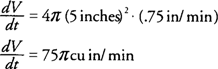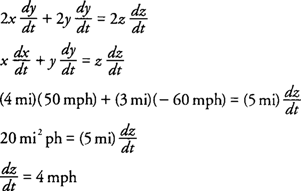## Related Rates of Change

Some problems in calculus require finding the rate of change or two or more variables that are related to a common variable, namely time. To solve these types of problems, the appropriate rate of change is determined by implicit differentiation with respect to time. Note that a given rate of change is positive if the dependent variable increases with respect to time and negative if the dependent variable decreases with respect to time. The sign of the rate of change of the solution variable with respect to time will also indicate whether the variable is increasing or decreasing with respect to time.

Example 1: Air is being pumped into a spherical balloon such that its radius increases at a rate of .75 in/min. Find the rate of change of its volume when the radius is 5 inches.

The volume ( V) of a sphere with radius r isDifferentiating with respect to t, you find thatThe rate of change of the radius dr/dt = .75 in/min because the radius is increasing with respect to time.

At r = 5 inches, you find thathence, the volume is increasing at a rate of 75π cu in/min when the radius has a length of 5 inches.

Example 2: A car is traveling north toward an intersection at a rate of 60 mph while a truck is traveling east away from the intersection at a rate of 50 mph. Find the rate of change of the distance between the car and truck when the car is 3 miles south of the intersection and the truck is 4 miles east of the intersection.

• Let x = distance traveled by the truck
• y = distance traveled by the car
• z = distance between the car and truck

The distances are related by the Pythagorean Theorem: x 2 + y 2 = z 2 (Figure 1) .Figure 1 A diagram of the situation for Example 2.

The rate of change of the truck is dx/dt = 50 mph because it is traveling away from the intersection, while the rate of change of the car is dy/dt = −60 mph because it is traveling toward the intersection. Differentiating with respect to time, you find thathence, the distance between the car and the truck is increasing at a rate of 4 mph at the time in question.